## Evaluating Limits

Limits of functions are evaluated using many different techniques such as recognizing a pattern, simple substitution, or using algebraic simplifications. Some of these techniques are illustrated in the following examples.

Example 1: Find the limit of the sequence:Because the value of each fraction gets slightly larger for each term, while the numerator is always one less than the denominator, the fraction values will get closer and closer to 1; hence, the limit of the sequence is 1.

Example 2: Evaluate.

When x is replaced by 2, 3 x approaches 6, and 3 x − 1 approaches 5; hence,.

Example 3: Evaluate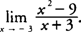.

Substituting −3 for x yields 0/0, which is meaningless. Factoring first and simplifying, you find that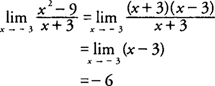The graph of (x 2 − 9)/(x + 3) would be the same as the graph of the linear function y = x − 3 with the single point (−3,−6) removed from the graph (see Figure 1).Figure 1 The graph of y = ( x 2 − 9)/( x + 3).

Example 4: Evaluate.

Substituting 3 for x yields 0/0, which is meaningless. Simplifying the compound fraction, you find that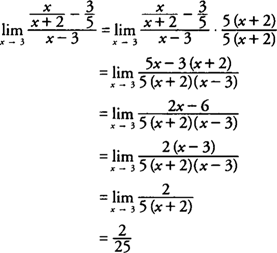Example 5: Evaluate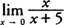.

Substituting 0 for x yields 0/5 = 0; hence,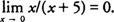Example 6: Evaluate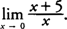Substituting 0 for x yields 5/0, which is meaningless; hence,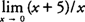DNE. (Remember, infinity is not a real number.)

Back to Top
A18ACD436D5A3997E3DA2573E3FD792A